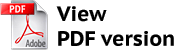https://vestnik.volbi.ru/
﻿

# OCCURRENCE OF A PLANE WAVE IN THE MAIN FACTORS OF PRODUCTION

Back to articles of current journal
 PDF:Author(s): Kuligin E. V., Kuznetsov S. B., Number of journal: 1(58) Date: February 2022 Annotation: Our task is to find the conditions for the occurrence of a plane wave in the main factors of production. The mathematical apparatus of vector analysis is the main tool for studying the behavior of production factors. A systematic analysis of changes in the growth of factors of production and a scientific-abstract method in the vector analysis of economic development were used to obtain the main results. The differential equation describing the occurrence of a plane wave in the development of factors of production was obtained in earlier works. On the basis of this equation, it was possible to obtain the conditions for the appearance of a plane wave, under the assumption of the potentiality of the investment field and the validity of the property of proportionality with respect to the rate of change of factors of production. A plane wave in the development of production factors arises when the vectors of the wave number and the rate of renewal of production factors are parallel. Another condition for the occurrence of a plane wave is the fulfillment of the condition: the angular frequency is half of the scalar product of the vectors of the wave number and the rate of renewal of production factors. Under a stationary development of the factors of production and the condition of no vortex, the dependence of the behavior of the modulus of the rate of change of the factors of production on the size of the economy is found. The mathematical apparatus proposed in the work makes it possible to determine the amount of government funding, and also allows you to find the proportions in which funding of the main factors of production is required. A sharp change in the proportions of investment in factors of production will lead to the emergence of a vortex, which can lead to instability in economic development. The use of this apparatus allows you to simulate the results of investment in the main factors of production and represents the practical significance of the work. Keywords: cyclicality of the economy, factors of production, flat wave, dynamic rotor, field potentiality, proportionality property, phase front, investment potentiality, wave number, vortex, circular frequency For citation: Kuznetsov S. B., Kuligin E. V. Occurrence of a plane wave in the main factors of production. Business. Education. Law, 2022, no. 1, pp. 82—89. DOI: 10.25683/VOLBI.2022.58.138.